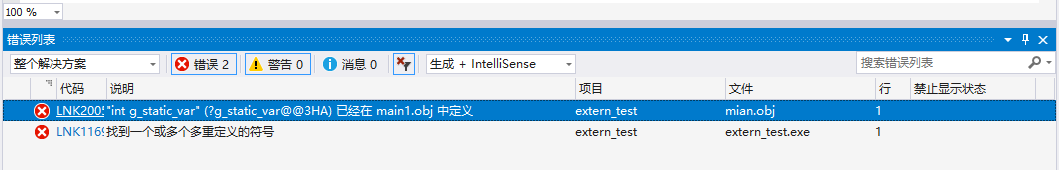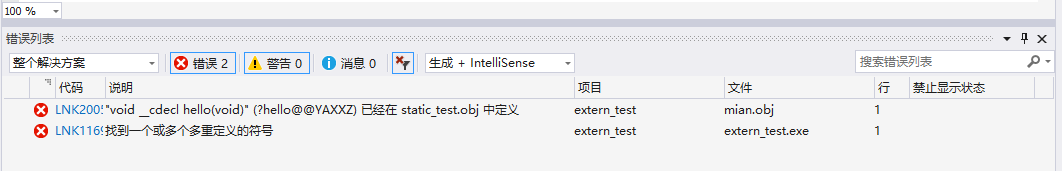A Programmer

# (原创)static关键字的作用

C++中的static关键字的作用，这里从两个方面来进行解析。一方面是放在变量前面时所产生的作用，另一方面就是放在函数前所产生的作用了。首先从static类型的变量开始。

## static修饰的变量

static修饰变量时，表明该变量是一个静态变量，静态变量存储在静态存储区内。静态存储区是内存在程序编译的时候就已经分配好，这块内存在程序的整个运行期间都存在。它主要存放静态数据、全局数据和常量。

### static修饰的全局变量

static修饰局部变量时，就表明这个变量是一个静态局部变量，这种变量一般在函数中用得比较多，可以用来记录函数的执行状态。例如下面的一个简单例子(编程环境Visual Studio 2017):

```#include <iostream>

using namespace std;

void static_var_test(void)
{
int i = 0;
static int j = 0;
cout << "i = " << i++ << "; j = " << j++ << endl;
}

int main(int argc, char **argv)
{
for (size_t i = 0; i < 10; i++)
{
static_var_test();
}

getchar();
}
```

```i = 0; j = 0
i = 0; j = 1
i = 0; j = 2
i = 0; j = 3
i = 0; j = 4
i = 0; j = 5
i = 0; j = 6
i = 0; j = 7
i = 0; j = 8
i = 0; j = 9
```

在这个例子中我们可以看到，在函数体内定义了一个局部变量，每当程序运行到该语句时都会给该局部变量分配栈内存。但随着程序退出函数体，系统就会收回栈内存，局部变量也相应失效。但有时候我们需要在两次调用之间对变量的值进行保存。通常的想法是定义一个全局变量来实现。但这样一来，变量已经不再属于函数本身了，不再仅受函数的控制，给程序的维护带来不便。

• 该变量在静态存储区分配内存；
• 静态局部变量在程序执行到该对象的声明处时被首次初始化，即以后的函数调用不再进行初始化；
• 静态局部变量一般在声明处初始化，如果没有显式初始化，会被程序自动初始化为0；
• 它始终驻留在静态存储区，直到程序运行结束。但其作用域为局部作用域，当定义它的函数或语句块结束时，其作用域随之结束；

### static修饰的全局变量

static修饰的全局变量和普通的全局变量相同的地方是其都是在静态存储区中分配内存。不同之处就在于变量的作用范围不同，无static修饰的全局变量是在整个程序的所有代码文件中都可以访问到的，而有static修饰的全局变量只能够在本文件内对其进行访问。下面我们通过一个例子来感受感受其区别，这里我们定义两个文件，main.cpp和main1.cpp，内容如下

```
// main.cpp
#include <iostream>

using namespace std;

int g_static_var = 1;

int main(int argc, char **argv)
{
cout << g_static_var << endl;
}

// main1.cpp
#include

using namespace std;

int g_static_var = 2;

int test1(void)
{
cout << g_static_var << endl;

return g_static_var;
}
``````
// extern.h
#pragma once

int g_var;
static int g_static_var;

// main1.cpp
#include <iostream>
#include "extern.h"

using namespace std;

int test1(void)
{
g_static_var = 20;

cout << g_static_var << endl;

return g_static_var;
}

// main.cpp
#include <iostream>
#include "extern.h"

using namespace std;

int main(int argc, char **argv)
{
g_static_var = 10;
cout << g_static_var << endl;
}

```### static修饰的类成员变量

和普通成员变量一样，静态成员变量也必须遵守public，protected和private访问规则。所不同的是，（1）对于非静态数据成员，每个类对象都有自己的拷贝。而静态数据成员被当作是类的成员。无论这个类的对象被定义了多少个，静态数据成员在程序中也只有一份拷贝，由该类型的所有对象共享访问。也就是说，静态数据成员是该类的所有对象所共有的。对该类的多个对象来说，静态数据成员只分配一次内存，供所有对象共用。所以，静态数据成员的值对每个对象都是一样的，它的值可以更新；（2）静态数据成员存储在静态存储区。静态数据成员定义时要分配空间，所以不能在类声明中定义；（3）因为静态数据成员在静态存储区中分配内存，属于本类的所有对象共享，所以，它不属于特定的类对象，在没有产生类对象时其作用域就可见，即在没有产生类的实例时，我们就可以操作它。下面举个例子：

```// static_test.h
#pragma once

class static_test {
private:
static int private_static_var;
int class_var;

public:
static int public_static_var;
static_test();
void print_class_var(void);
void print_static_var(void);
};

// static_test.cpp
#include "static_test.h"
#include <iostream>

int static_test::private_static_var = 10;
int static_test::public_static_var = 20;

static_test::static_test():class_var(30) {}

void static_test::print_class_var()
{
std::cout << "class_var = " << class_var << std::endl;
}

void static_test::print_static_var()
{
std::cout << "private_static_var = " << private_static_var << std::endl;
std::cout << "public_static_var = " << public_static_var << std::endl;
}

// main.cpp
#include "static_test.h"
#include <iostream>

int main(int argc, char **argv)
{
std::cout << "static_test::private_static_var = " << static_test::public_static_var << std::endl;
static_test::public_static_var = 100;
static_test test;
test.print_class_var();
test.print_static_var();

getchar();
}
```

• 因为静态成员变量存储在静态存储区内，而且其是在类的所有对象中共享的。所以它不属于特定的类，即时没有产生类的实例对象，我们也可以访问它
• 正是因为类静态成员变量是不属于任何实例对象的，所以类的静态成员变量必须在类外进行显式初始化
• 访问类静态成员变量，既可以通过类实例，也可以通过类名加作用域解释符进行

## static修饰的函数

### static修饰的全局函数

static修饰的全局函数和static修饰的全局变量类似，其作用也是改变函数的链接性，如果全局函数中，没有static修饰的话，那么这个函数的链接性就是全局的，意味着其他文件中也可以访问到这个函数，只要用extern该函数是在别处定义的即可。但是如果该全局函数使用了static修饰的话，那么就代表该函数的链接性只局限于本文件，其他文件是无法访问或者调用该函数的。下面一个例子看看，创建两个文件main.cpp和static_test.cpp:

```
// main.cpp
#include "static_test.h"
#include <iostream>

void hello(void)
{
std::cout << "Hello World!" << std::endl;
}

static void hello2(void)
{
std::cout << "Static Hello World!" << std::endl;
}

int main(int argc, char **argv)
{

}

// static_test.cpp
#include "static_test.h"
#include <iostream>

void hello(void)
{
std::cout << "Hello World!" << std::endl;
}

void hello2(void)
{
std::cout << "Static Hello World!" << std::endl;
}

```• 静态函数只限于本文件内使用，不用害怕会被其他文件使用
• 多个文件可以定义函数名相同，但是函数体不同的同名全局函数

### static修饰的类成员函数

与静态数据成员一样，我们也可以创建一个静态成员函数，它为类的全部服务而不是为某一个类的具体对象服务。静态成员函数与静态数据成员一样，都是类的内部实现，属于类定义的一部分，所以我们也可以直接通过类调用静态成员函数，即时类对象还没有创建过。此外普通的成员函数一般都隐含了一个this指针，this指针指向类的对象本身，因为普通成员函数总是具体的属于某个类的具体对象的。通常情况下，this是缺省的。如函数fn()实际上是this->fn()。但是与普通函数相比，静态成员函数由于不是与任何的对象相联系，因此它不具有this指针。从这个意义上讲，它无法访问属于类对象的非静态数据成员，也无法访问非静态成员函数，它只能调用其余的静态成员函数。下面的例子是修改自前面静态类成员一节的例子而来：

```
// static_test.h
#pragma once

class static_test {
private:
static int private_static_var;
int class_var;

public:
static int public_static_var;
static_test();
void print_class_var(void);
void print_static_var(void);
static void print_all(void);    // 添加此static成员方法
};

// static_test.cpp
#include "static_test.h"
#include <iostream>

int static_test::private_static_var = 10;
int static_test::public_static_var = 20;

static_test::static_test():class_var(30) {}

void static_test::print_class_var()
{
std::cout << "class_var = " << class_var << std::endl;
}

void static_test::print_static_var()
{
std::cout << "private_static_var = " << private_static_var << std::endl;
std::cout << "public_static_var = " << public_static_var << std::endl;
}

void static_test::print_all()
{
std::cout << "private_static_var = " << private_static_var << std::endl;
std::cout << "public_static_var = " << public_static_var << std::endl;
}

// main.cpp
#include "static_test.h"
#include <iostream>

int main(int argc, char **argv)
{
std::cout << "static method: " << std::endl;
static_test::print_all();

std::cout << "static_test::public_static_var = " << static_test::public_static_var << std::endl;
static_test::public_static_var = 100;
static_test test;
test.print_class_var();
test.print_static_var();

getchar();
}

```

• 在类外定义函数时，不能带static，否则会报错
• 静态成员方法里面只能访问类的静态成员变量，因为静态类成员方法不属于任何类对象，可以在对象创建前就被调用，此时类的其他成员变量都还没有被创建，当然是不能被访问到的
• 反过来，非静态成员方法确实可以访问静态成员变量的，这个不受限制